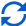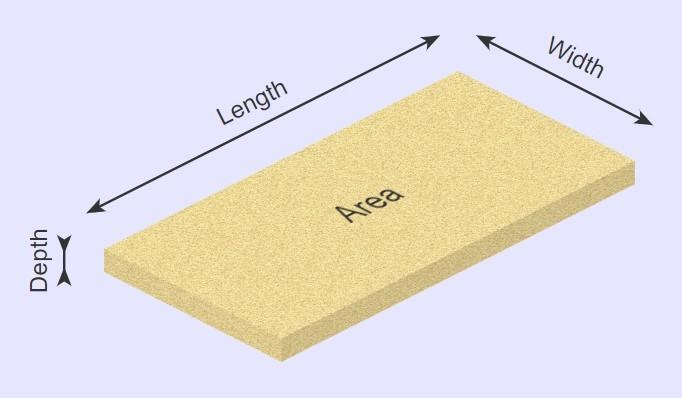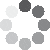Enter parameters LENGTH, WIDTH & DEPTH
Enter parameters
LENGTH, WIDTH & DEPTH
Length:
ft
• inches (in)
• feet (ft)
• yards (yd)
• centimeters (cm)
• meters (m)
Width:
ft
• inches (in)
• feet (ft)
• yards (yd)
• centimeters (cm)
• meters (m)
Depth:
in
• inches (in)
• feet (ft)
• yards (yd)
• centimeters (cm)
• meters (m)
Density:
100 - Sand lb/ft³
• Sand - 100 lb/ft³
• Custom
Price per unit of mass:
t
• pound (lb)
• US short ton (t)
• imperial-long ton (long t)
• kilogram (kg)
Price per unit of volume:
yd³
• cubic yards (yd³)
• cubic feet (ft³)
• cubic inches (in³)
• cubic centimeters (cm³)
• cubic meters ()Result
Area:
0
ft²
• square feet (ft²)
• square inches (in²)
• square yards (yd²)
• square miles (mi²)
• acres (ac)
• square centimeters (cm²)
• square meters ()
Volume:
0
yd³
• cubic yards (yd³)
• cubic feet (ft³)
• cubic inches (in³)
• cubic centimeters (cm³)
• cubic meters ()
Weight:
0
t
• pounds (lb)
• US short tons(t)
• imperial-long tons (long t)
• kilograms (kg)
Cost:
$Enter parameters AREA & DEPTH Area: ft² • square feet (ft²) • square inches (in²) • square yards (yd²) • square miles (mi²) • acres (ac) • square centimeters (cm²) • square meters () Depth: in • inches (in) • feet (ft) • yards (yd) • centimeters (cm) • meters (m) Density: 100 - Sand lb/ft³ • Sand - 100 lb/ft³ • Custom Price per unit of mass: t • pounds (lb) • US short tons (t) • imperial-long tons (long t) • kilograms (kg) Price per unit of volume: yd³ • cubic yards (yd³) • cubic feet (ft³) • cubic inches (in³) • cubic centimeters (cm³) • cubic meters ()Result Volume: 0 yd³ • cubic yards (yd³) • cubic feet (ft³) • cubic inches (in³) • cubic centimeters (cm³) • cubic meters () Weight: 0 t • pounds (lb) • US short tons (t) • imperial-long tons (long t) • kilograms (kg) Cost:$
Enter parameters
VOLUME
Volume:
yd³
• cubic yards (yd³)
• cubic feet (ft³)
• cubic inches (in³)
• cubic centimeters (cm³)
• cubic meters ()
Density:
100 - Sand lb/ft³
• Sand - 100 lb/ft³
• Custom
Price per unit of mass:
t
• pounds (lb)
• US short tons (t)
• imperial-long tons (long t)
• kilograms (kg)
Price per unit of volume:
yd³
• cubic yards (yd³)
• cubic feet (ft³)
• cubic inches (in³)
• cubic centimeters (cm³)
• cubic meters ()Result
Weight:
0
t
• pounds (lb)
• US short tons (t)
• imperial-long tons (long t)
• kilograms (kg)
Cost:
$(1 votes, average: 5.00 out of 5)Loading... Similar Calculators: Real-time graphics. Make the calculations and see the changes. Real-time graphics. Make the calculations and see the changes. Real-time graphics. Make the calculations and see the changes. Embed Similar Calculators:Calculating how much sand your landscaping project will need is not easy. It can be difficult to look at a space and estimate how much is required, and this can result in wasted money and time. That’s why we built our sand calculator. Use it to calculate the volume of sand you will need, the weight of the material, and if you know the price per unit mass/volume, then the total cost as well. Contents: ## Sand calculator formulaWhen you enter your measurements into the calculator, it works out the area and volume of the sand required using the below formulas: $$Area = Length \times Width$$ $$Volume = Area \times Depth$$ In addition If you know the density of the material you are using (NB: standard sand is 100 lb/ft³), the sand estimator can work out how many tons of sand you will require using the formula: $$Weight = Volume \times Density$$ Want to know the best part? If you have the price per unit mass (e.g. cost per pound) or cost per unit volume (e.g. cost per cubic feet), then the calculator can work out the total cost of the required sand: $$Cost = Price\,per\,unit\,mass \times Weight$$ or $$Cost = Price\,per\,unit\,volume \times Volume$$ ## Example calculations • Length, Width and Depth with Price Per Unit Mass Imagine I am going to fill an area measuring 5 feet by 3 feet using standard sand. I want equal coverage to a thickness of 2 inches. The sand’s density is 100 lb/ft³ and costs$10 per ton.

The calculator would perform the following calculations:

$$Area = Length \times Width = 5 ft \times 3 ft = 15 ft^2$$

$$Volume = Area \times Depth = 15 ft^2 \times 2\,in = 2.5 ft^3$$

$$Weight = Volume \times Density = 2.5ft^3 \times 100\,lb/ft^3 = 0.125\,t$$

$$Cost = Price\,per\,unit\,mass \times Weight = 10\,/t \times 0.125\,t= 1.25$$

• Rectangular Area and Depth with Price Per Unit Volume

Let’s say I have an area of 100 ft²  that I want to cover with sand to a depth of 3 inches.

The sand’s density is 100 lb/ft³  and costs $15 per cubic yard. The calculator would perform the following calculations: $$Volume = Area \times Depth = 100 ft^2 \times 3\,in = 25\,ft^3$$ $$Weight = Volume \times Density = 25ft^3 \times 100\,lb/ft^3 = 2,500\,lb$$ $$Cost = Price\,per\,unit\,volume \times Volume = 15\,/yd^3 \times 2,500\,lb = 13.89$$ At this point, you’ll likely be asking: What if I don’t know the density? What if I’m using a different material? 🤔 Well, our calculator has an option for “custom density”, which you can calculate using the following formula: $$Density = {Mass \over Volume}$$ We will show you how to use this formula in our final example. • Volume With Custom Density Let’s imagine that I want to fill a space measuring 40 ft³ with a custom aggregate costing$20 per ton.

I am unsure of its density, but when buying by bulk the sand weighs 240 pounds per 4 cubic feet.

I can therefore calculate its density:

$$Density = {Mass \over Volume} = {240\,lb \over 4\,ft^3} = 60\,lb/ft^3$$

I enter these values in the calculator.

$$Weight = Volume \times Density = 40\,ft^3 \times 60\,lb/ft^3 = 2,400\,lb$$

$$Cost = Price\,per\,unit\,mass \times Weight = 20\,/t \times 2,400\,lb = 24$$

## What if my measurements aren’t in feet and pounds?

You may have noticed that we switch between units.

For example, when calculating the amount of sand and cost in the last example, price is given in dollars per ton and weight is given in pounds.

There are multiple options for length, density, mass and volume.

Our calculator does the conversions for you using the pre-programmed conversions:

$$1\,foot = 12\,inches = 0.33\,yards = 30.48\,centimeters = 0.3048\,meters$$

$$1\,US\,short\,ton = 2000\,pounds = 0.893\,imperial\,long\,ton = 907\,kg$$

It’s that simple! 😉

Embed this calculator on your site!Add live graphics
Copied to clipboard! Preview